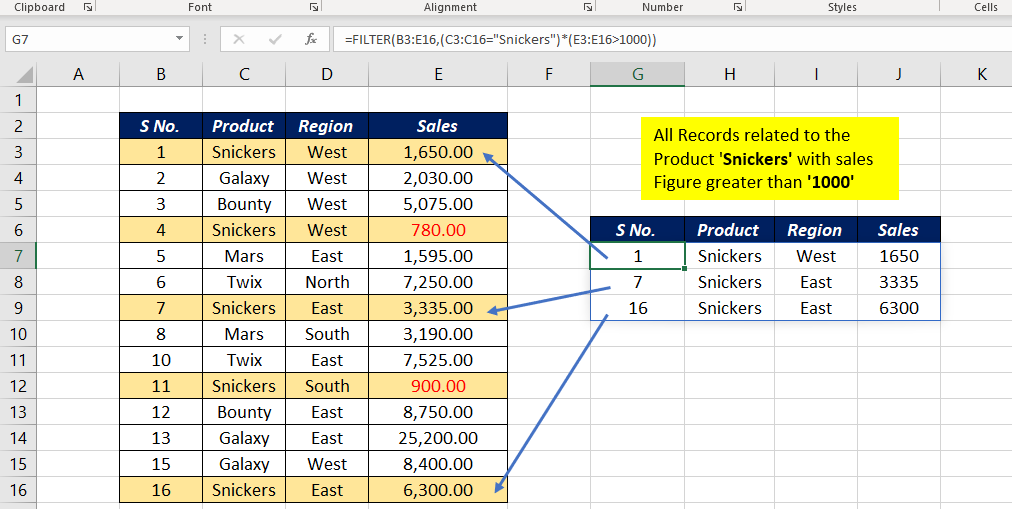# FILTER FunctionThe Excel FILTER function is a DYNAMIC ARRAY FUNCTION that can filter a data range and return an array of values based on the conditions specified.

The result is an array of matching values and these will values will ‘spill’ on to the worksheet. As FILTER function is dynamic, when values in the source data change, or the source data array is resized, the spill range will update automatically.

### Function Type

Lookup and reference

### Purpose

Filter a data range for single or multiple conditions

### Return value

An array of filtered values

### Syntax

`=FILTER (array, include, [if_empty])`

### Arguments

array – Array or range to filter

include – a logical test that returns the Boolean array (Criteria)

if_empty – [optional] Value to return if the filter results in no records

## Examples

#### Example 1

In this example, FILTER function is used to extract all records from the data range B3:E16, related to the product ‘Mars‘.

`=FILTER(B3:E16,C3:C16="Mars")`

#### Example 2

In the above example, the direct value ‘Mars’ is used to define the condition. In this example, a cell reference (H3) is used in the place of the second argument.

The following formula will filter the records for the condition specified in the cell H3. In this case, ‘Snickers‘ is the value in the cell H3 and all records related to the product called Snickers are filtered.

`=FILTER(B3:E16,C3:C16=H3)`

#### Example 3

If the values or records satisfying the conditions are not found, the FILTER function will return a #CALC! error.

To avoid this error, use the third argument if_empty

`=FILTER(B3:E16,C3:C16=H3,"Not Found")`

The above formula will return the text ‘Not Found’ if the function can’t find any record matching the specified criteria.

#### Example 4

An example of the FILTER function where multiple conditions are used.

Use the following formula to filter all records related to the product ‘Snickers’, whose sales figures are ‘greater than 1000‘.

`=FILTER(B3:E16,(C3:C16="Snickers")*(E3:E16>1000))`